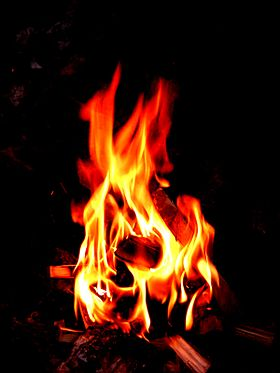# 2.9: Some Simple Patterns of Chemical Reactivity

Learning Objectives

• To introduce the basic patterns of chemical reaction.

There is an underlying explanation of why a reaction takes place from an underlying chemical theory based on chemical reactivity. In this sections, we discussed various reactions to identify key chemical trends that allows chemists to predict the outcome of chemical reactions. By recognizing general patterns of chemical reactivity, you will be able to successfully predict the products formed by a given combination of reactants We can often predict a reaction if we have seen a similar reaction before. For example, sodium ($$\ce{Na}$$) reacts with water ($$\ce{H_2O}$$) to form sodium hydroxide ($$\ce{NaOH}$$) and $$\ce{H_2}$$ gas:

$\ce{ 2Na(s) + 2H2O(l) -> 2NaOH(aq) + H2(g)} \nonumber$

with $$\ce{(aq)}$$ indicating aqueous liquid. As discussed later, potassium ($$\ce{K}$$) is in the same family (column) of elements as sodium and exhibits similar chemistry. Therefore, one might predict that the reaction of $$\ce{K}$$ with $$\ce{H2O}$$ would be similar to that of $$\ce{Na}$$:

$\ce{2K(s) + 2H2O(l) -> 2KOH(aq) + H2(g)} \nonumber$

In fact, all alkali metals react with water to form their hydroxide compounds and hydrogen.

## Combination and Decomposition Reactions

In combination reactions, two or more compounds react to form a single, more complex compound. Many elements react with one another in this fashion to form compounds. The general chemical equation for combination reaction is:

$\ce{ A + B -> C} \nonumber$

and an example includes the generation of ammonia ($$\ce{NH_3}$$) from nitrogen ($$\ce{N_2}$$), and hydrogen ($$\ce{H_2}$$):

$\ce{ N2(g) + 3H2(g) -> 2NH3(g)} \nonumber$

In decomposition reactions one substance undergoes a reaction to form two or more simpler products. Such reactions often occur when compounds are heated or electricity is added. The general chemical equation for decomposition reaction is:

$\ce{A -> B + C } \nonumber$

For example, the thermal decomposition of limestone ($$\ce{CaCO3(s)}$$) generates quicklime ($$\ce{CaO(s)}$$) and carbon dioxide $$\ce{CO2(g)}$$

$\ce{CaCO3(s) -> CaO(s) + CO2(g)} \nonumber$

## Combustion in Air

Combustion reactions are rapid reactions that produce a flame. Most common combustion reactions involve oxygen (O2) from the air as a reactant. For example, wood will combust in the presence of oxygen in that air (with an ignition event) to generate a fire.Figure $$\PageIndex{1}$$: The flames caused as a result of a fuel undergoing combustion (burning) (CC BY-SA 2.5; Et bål via Wikipedia)

A common class of compounds which can participate in combustion reactions are hydrocarbons (compounds that contain only carbon and hydrogen).

Table $$\PageIndex{1}$$: Examples of common hydrocarbons
Hydrocarbon Molecular formula
methane CH4
propane C3H8
butane C4H10
octane C8H18

When hydrocarbons are combusted they react with oxygen ($$\ce{O_2}$$) to form carbon dioxide ($$\ce{CO_2}$$) and water ($$\ce{H_2O}$$). For example, when propane is burned the reaction is:

$\ce{ C3H8(g) + 5O2(g) -> 3CO2(g) + 4H2O(l)} \label{3.2.6}$

Other compounds which contain carbon, hydrogen and oxygen (e.g. the alcohol methanol CH3OH, and the sugar glucose C6H12O6) also combust in the presence of oxygen ($$\ce{O_2}$$) to produce $$\ce{CO_2}$$ and $$\ce{H_2O}$$.